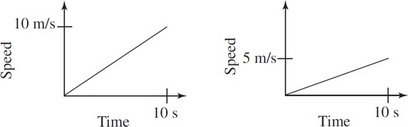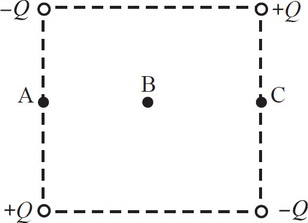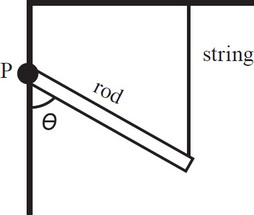# AP Physics 1 Practice Test 2

### Test Information10 questions18 minutes

1. A horizontal spring-block oscillator is in motion when a second block of equal mass falls on top of the oscillating block. If the two blocks stick together, what effect will this have on the amplitude of the oscillations?

2.The graphs above show the changes in speed for two different blocks. The left graph shows the speed over time of Block 1, which has a mass of m1, and the right graph shows the speed of Block 2, which has a mass of 2m1. While speeding up, they both had to overcome some frictional force, f. Which of the two blocks had more power provided to it?

3. If the force of gravity between the Moon and the Earth were to stop, which statement best describes the resulting motion of the moon?

4. The weight of a box on the Earth is 80 N. What is the weight of the box on the moon if the moon has 1/80th the mass and 1/4th the radius of the Earth?

5. A ball of mass is attached to a string connected to the ceiling, forming a pendulum. When the string is raised to an angle of 50 degrees below the horizontal (the ceiling) and released, the ball swings back and forth with a period of 0.4 s. Which of the following changes to the pendulum would decrease the period of the pendulum?

6. Three light bulbs, each with a resistance of R, are connected in parallel to a battery in a simple circuit. If the middle bulb goes out, then

7.The figure above shows a plane diagram. The figure shows four point charges arranged at the corners of a square with point B as its center. A positive charge would experience no net force if it were placed at which of the three points shown in the figure?

8. A beam of monochromatic light entering a glass window pane from the air will experience a change in

9.If the rod in the figure above is uniform and has mass m, what is the tension in the supporting string? The rod is free to rotate about point P.

10. An ideal battery of constant voltage V supplies current to a circuit that has total resistance R. If R is doubled, what will happen to the total power dissipated by the circuit?